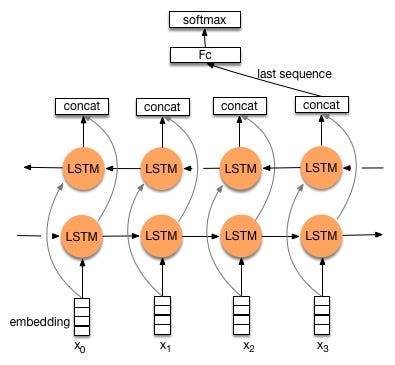## Lecture 8: sequence classification

• sequence classification
• sequence prediction
• seq2seq(https://www.csdn.net/article/2015-08-28/2825569)
• Input: sentence
• Output: sentence
• pinput -> intermediate state -> output (see the reference)
• encoder:
• decoder:
• Bidirectional LSTM
•## The key of using Unity Texture array

1. The texture array structure in different from GLSL and Unity + HLSL, the sequence of image index i and j must be adjusted. Suppose the light field has structure 16 x 16:

2. The program of using texture array in unity
1. Suppose we want to load the images in folder “lytro”, we must mkdir called “Resources” in the folder “Asset”, then drag the folder “lytro” into “Resources”.

## Word2Vec Models

Collection of all pre-trained Word2Vec Models:

http://ahogrammer.com/2017/01/20/the-list-of-pretrained-word-embeddings/

Here are some similarity tests of Google’s model:

The similarity between good and great is: 0.7291509541564205
The similarity between good and awesome is: 0.5240075080190216
The similarity between good and best is: 0.5467195232933185
The similarity between good and better is: 0.6120728804252082
The similarity between great and awesome is: 0.6510506701964475
The similarity between great and best is: 0.5216033921316416
The similarity between great and better is: 0.43074460922502006
The similarity between awesome and best is: 0.3584938663818339
The similarity between awesome and better is: 0.27186951236001483
The similarity between best and better is: 0.5226434484898708
The similarity between food and foodie is: 0.3837408842876883
The similarity between food and eat is: 0.5037572298482941
The similarity between foodie and eat is: 0.3050075692941569
The similarity between park and view is: 0.1288395798972001
The similarity between design and art is: 0.3347430713890944

## Lecture 8: Evaluation

• PCFG
• ∑Pr(A -> gamma | A) = 1
• (conditional) probability of each item has to sum to one
• Pr(O = o1,o2,…,on|µ)
• HMM: Forward
• PCFG: Inside-Outside
• Guess Pr: argmax_(Z)[ Pr(Z|O, µ) ]
• HMM:Use Viterbi to get
• PCFG: Use Viterbi CKY to get
• *Z is the best sequence of states
• Guess µ: argmax_(µ)[Pr(O|µ)]
• HMM:Use forward-backward to get
• PCFG: Use Inside-outside to get
• Example:
• Sentence:
• ——————-S
• ——–NP—————-VP
• ——–NP———-V————-NP
• —————————roasted—-peanuts
• Problem:
• Pr_µ(peanuts eat roasted people) = Pr_µ(people eat roasted peanut)
• We can try to generate head of each phrase:
• Dependency representation:
• Sentence:
• —————————eat
• —————people—————peanuts
• —————–the—————–roasted
• Lexical (bottom up)
• NP ->det N
• Evaluation
• Reference Reading:How Evaluation Guides AI Research
• Intrinsic evaluation
• Extrinsic evaluation
• Kappa’s evaluation
• Metric: precision recall
• How to evaluate two structures which could generate the same sentence?
• Answer: Generate more than one output for each input, convert the output into set of output, and use precision and recall to measure.
• If the reader’s score agree with the machine, stop

## 03102018

• Gensim Tutorial:
• Use google-news model as pre-trained model
• clustering based on distance matrix
• Question: how do we do the clustering?
• should cluster on the keywords?
• should cluster on the keywords-related words?
• Leg dissection demo:
• 18 cameras 30frames 10G
• 5 cameras 100 frames 6G
• Question:
• we cannot change focal length now. we can only change the viewpoint
• if we want dynamic, we should have dynamic mesh?
• Foveated ray-tracing:
• input: eye ray + 1spp
• output: foveated image
• question: If we use foveated image as ground truth, what should be the denoising algorithm for the ground truth?
• TODO:
• read G3D code and change sample number
• Homework

## Lecture 6: Context-free parsing

Questions:

1. Generative Model P(X,Y)
2. Discriminative model P(Y|X)

MainPoints

1. Block sampler: Instead of sample one element at a time, we can sample a batch of samples in Gibbs Sampling.
2. Lag and Burn-in: can be viewed as parameters (we can control the number of iterations)
1. lag: mark some iterations in the loop as lag, then throw away the lag iterations, then the other samples become independent.
1. Example: run 1000 iters -> run 100 lags -> run 1000 iters -> 100 lags …
2. burn in: throw away the initial 10 or 20 iterations (burn-in iterations), where the model has not converged.
1. The right way is to test whether the model has converged.
3. Topic model:
4. The sum of the parameter of each word in a topic doesn’t need to be one
5. The derivative (branches) of LDA (Non-parametric model):
1. Supervised LDA
2. Chinese restaurant process (CRP)
3. Hierarchy models
1. example: SHLDA
2. gun-safety (Clinton) &  gun-control (Trump)

Parsing:

Any context which can be processed with FSA can be processed with CFGs. But not vice versa.

 ? turning machine (Don’t cover in this lecture) CSL Tree adjusting Grammar PTAGs (Don’t cover in this lecture) CF PDA/CFGs PCFGs PDA/CFGs Allow some negative examples. And can handle some cases that cannot be processed by FSA. For example: S -> aSb {a^nb^n} cannot be processed by FSA because we need to know the variable n. But FSM only remember the states, it cannot count. Regular FSA/regular expressions HMM

Example1:

The rat that the cat that the dog bit chased died.

Example2:

Sentence: The tall man loves Mary.

————-loves

—–man————Mary

-The——tall

Structure:

——————-S

——–NP——————VP

—the-tall–man       loves—–Mary

Example3: CKY Algorithm

0 The 1 tall 2 man 3 loves 4 Mary 5

[w, i, j] A->w \in G

[A, i, j]

Chart (bottom up parsing algorithm):

0 The 1 tall 2 man 3 loves 4 Mary 5

–Det —— N ———V ——NP—-

———–NP ———–VP ———

—————- S ———————

Then I have:

[B, i, j]

[C, j, k]

So I can have:

A->BC : [A, i, k] # BC are non-determinal phrases

NP ->Det N

VP -> V NP

S -> NP VP

Example4:

I      saw         the        man       in        the       park        with      a       telescope.

——————– NP—————– PP ———–

–                         —————NP———————

————————NP—————————–

A->B . CD

CD: predicted

[A -> a*ß, j]

[0, S’-> *S, 0]

scan: make progress through rules.

[i, A -> a* (w_j+1) ß, j]

A [the tall] B*C

i, [the tall], j

Prediction: top-down prediction B-> γ

[i, A-> a * Bß, j]

[j, B->*γ, j]

Combine

Complete (move the dot):

[i, A->a*ß, k] [k, B->γ, j]

I                          k                       j

–[A->a*Bß]———[B->γ*]—–

Then I have:

[I, A->aB*ß, k]

## Lecture 5: Reduced-dimensionality representations for documents: Gibbs sampling and topic models

• watch the new talk and write summary
• Noah Smith: squash network
• Main points:
• difference between LSA & SVD
• Bayesian graphical models
• informative priors are useful in the model
• Bayesian network
• DAG
• X1X2…Xn
• Po(X1, X2, …, Xn)
• Generative story: HMM (dependencies)
• A and B are conditionally independent given C iff P(A,B|C) = P(A|C) * P(B|C)
•       C (to A, to B)
•   A                             B
• Example:
•               Cloudy (to Sprinter and rain)
• Sprintder(to wet grass)                   Rain (to wet grass)
•                       Wet grass(final state)
•w’s are observable
• State change graph:  π -> S1 -> S2 -> S3 -> … -> St
• w’s are observable
• μ~(A,B,π)
• Have a initial π for C, π~Beta(Gamma(π1), Gamma(π2))
• C is Bernoulli (π)
• S~ Bernoulli(π(S | C))
• R~Bernoulli(π(S | C))
• W ~ Bernoulli(\tao (ω | S,R))
• π~Dirichlet(1)
• S1~Cat(π)S2~Cat(a_s1,1  ,  a_s1,2  ,   ….  ,   a_(s1,n))* Cat is chosen from the transition matrix
• ω1~Cat(b_s1,1  ,  b_s1,2  ,   ….  ,   b_(s1,n))ω2~Cat(b_s1,1  ,  b_s1,2  ,   ….  ,   b_(s1,n))* Cat is chosen from the transition matrix
• What just been introduced is the unigram model, here is the bigram model
•• P(s1, s2, …. , sn) = P(s1) P(s2|s1) P(s3|s1,s2) P(s4|s2,s3)..
• For EM, we need to recalculate everything, conditional distribution are different
• Some distributions:
• Binomial vs. Bernoulli
• Multinomial vs. discrete / categorical
• Document squashing

GLuint posLength = sizeof(PointStruct) * PointCloudData.size(); correct

GLuint posLength = sizeof(PointCloudData) ; wrong

glBufferData(GL_ARRAY_BUFFER, posLength, &PointCloudData, GL_STATIC_DRAW);

## Hello

• Cross entropy
• H(p,q) = D(p||q) + H(p)
• H(p) is some inherent randomness in p
• D(p||q) is what we care about. we can try to get D(p||q) by calculating cross entropy.
• Conclusion: a model is good is that it assign good approximation to the observed data. So we need to find some good q
• Main points:
• Example: She loves her. (It’s a correct string, but English is not like this. It should be “She loves herself.”)
• We need a meaning pair.
• Two orthogonal dimensions:
• probability for the strings.
•  Units Prob String {anbn|n>=1} P(w1, w2,…, wn) Structure A tree structure PCFG
• L1 = L2: Language 1 is equal to Language 2
• Weak equivalence
• Sense of string are the same.
• Strong equivalence
• Structure of language 1 and 2 are the same.
• G1 = G2 iff {x| G1 generates string x} = {x|G2 generates string x} (all and only the same structures)
•  G1 G2 S->a s s->s a s->e s->e
• G1 and G2 are weak equivalent (they generate the same strings) but not strong equivalent
• Example: Jon loves mary
• Questions:
• How to measure equivalence?
• binary judgements?
• EM
• Question: How to find a good model? Expectation maximization (EM)
• The structure of model is given, we need to find the parameters for the model.
• Coin: H H H H T T T T T T
•• MLE: argmax [p(x|mu)]
•• Solve:• Result: p = k/n
• HMM <A,B, pi>